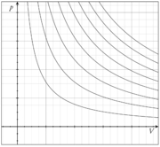xIdeal gas lawOverview

The ideal gas law is the equation of state
Equation of state
In physics and thermodynamics, an equation of state is a relation between state variables. More specifically, an equation of state is a thermodynamic equation describing the state of matter under a given set of physical conditions...

of a hypothetical ideal gas
Ideal gas
An ideal gas is a theoretical gas composed of a set of randomly-moving, non-interacting point particles. The ideal gas concept is useful because it obeys the ideal gas law, a simplified equation of state, and is amenable to analysis under statistical mechanics.At normal conditions such as...

. It is a good approximation to the behavior of many gas
Gas
Gas is one of the three classical states of matter . Near absolute zero, a substance exists as a solid. As heat is added to this substance it melts into a liquid at its melting point , boils into a gas at its boiling point, and if heated high enough would enter a plasma state in which the electrons...

es under many conditions, although it has several limitations. It was first stated by Émile Clapeyron
Benoit Paul Émile Clapeyron
Benoît Paul Émile Clapeyron was a French engineer and physicist, one of the founders of thermodynamics.-Life:...

in 1834 as a combination of Boyle's law
Boyle's law
Boyle's law is one of many gas laws and a special case of the ideal gas law. Boyle's law describes the inversely proportional relationship between the absolute pressure and volume of a gas, if the temperature is kept constant within a closed system...

and Charles's law
Charles's law
Charles' law is an experimental gas law which describes how gases tend to expand when heated. It was first published by French natural philosopher Joseph Louis Gay-Lussac in 1802, although he credited the discovery to unpublished work from the 1780s by Jacques Charles...

. It can also be derived from kinetic theory
Kinetic theory
The kinetic theory of gases describes a gas as a large number of small particles , all of which are in constant, random motion. The rapidly moving particles constantly collide with each other and with the walls of the container...

, as was achieved (apparently independently) by August Krönig
August Krönig
August Karl Krönig was a German chemist and physicist who published an account of the kinetic theory of gases in 1856, probably after reading a paper by John James Waterston....

in 1856 and Rudolf Clausius
Rudolf Clausius
Rudolf Julius Emanuel Clausius , was a German physicist and mathematician and is considered one of the central founders of the science of thermodynamics. By his restatement of Sadi Carnot's principle known as the Carnot cycle, he put the theory of heat on a truer and sounder basis...

in 1857.

The state
State function
In thermodynamics, a state function, function of state, state quantity, or state variable is a property of a system that depends only on the current state of the system, not on the way in which the system acquired that state . A state function describes the equilibrium state of a system...

of an amount of gas
Gas
Gas is one of the three classical states of matter . Near absolute zero, a substance exists as a solid. As heat is added to this substance it melts into a liquid at its melting point , boils into a gas at its boiling point, and if heated high enough would enter a plasma state in which the electrons...

is determined by its pressure, volume, and temperature.